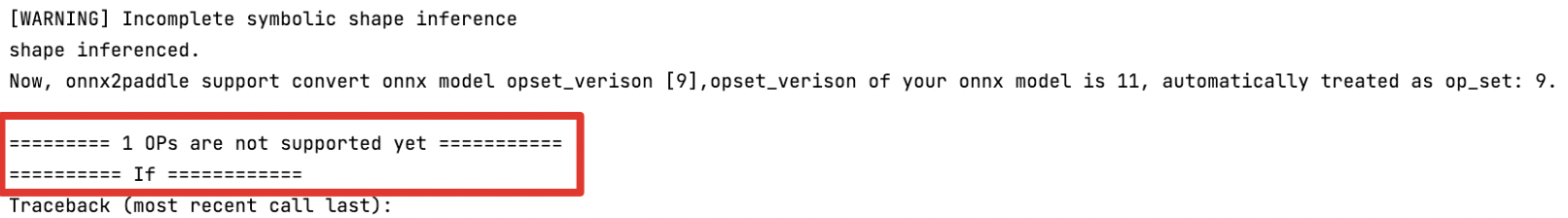## 一、概述¶

### 1.1 支持迁移的源模型¶

• Pytorch、ONNX、TensorFlow、Caffe 模型，可以通过 X2Paddle 直接完成模型转换，各框架支持的版本请参见下文 1.4 迁移环境依赖

• 其他框架如 MXNet、MindSpore 等，可以先转为 ONNX 格式的模型，再使用 X2Paddle 进行转换。

### 1.2 支持迁移的目标模型¶

• 服务器端，可以通过 X2Paddle 直接转换为 Paddle Inference 支持的格式，即 `.pdmodel``.pdiparams``.pdiparams.info` 格式文件。

• 移动/IoT 端，可以通过 X2Paddle 直接转换为 Paddle Lite 支持的格式，即 `.nb` 格式文件。

### 1.3 迁移工具和用法¶

#### 1.3.2 使用 Python API 迁移¶

```from x2paddle.convert import pytorch2paddle

# 省略模型定义和参数加载的代码
torch_module.eval()
save_dir="./pd_model",
jit_type="trace",
input_examples=[torch_input])
enable_code_optim=False,
convert_to_lite=False,
lite_valid_places="arm",
lite_model_type="naive_buffer")
```

（1）模型迁移配置说明

Pytorch 模型的迁移主要需要获取 Module 组网，加载模型参数 `state_dict()`，然后切换到 `eval()` 模式进行转换，使用默认参数下将转换为 Paddle Inference 的模型格式，通过 `convert_to_lite` 参数设置是否转换为 Paddle Lite 的模型格式。

• Pytorch 模型迁移需要准备 Module 组网代码和训练好的 `.pth` 模型参数文件；

• ONNX 模型迁移需要准备 `.onnx` 模型文件；

• Tensorflow 模型迁移需要准备 `.pb` 模型文件；

• Caffe 模型迁移需要准备 `.prototxt` 模型文件和 `.caffemodel`模型参数文件。

（2）模型迁移过程说明

module PyTorch 的 Module。
save_dir 转换后模型保存路径。
jit_type 转换方式，对应 Pytorch 中定义的两种模型转换方式 tracescript。默认为 trace。"trace" 方式生成的代码可读性较强，较为接近原版 PyTorch 代码的组织结构，支持大部分模型，但是不支持模型输入是动态 shape 的情况；"script" 方式不需要知道输入数据的类型和大小即可转换（支持动态 shape），使用上较为方便，但目前 PyTorch 支持的 script 代码方式有所限制，可能存在一些 op 和语法不支持。优先推荐使用 "trace" 方式。
input_examples torch.nn. Module 的输入示例，list 的长度必须与输入的长度一致。默认为 None。jit_type 为 "trace" 时，input_examples 不可为 None，转换后自动进行动转静；jit_type 为 "script" 时，当 input_examples 为 None 时，只生成动态图代码；当 input_examples 不为 None 时，才能自动动转静。
convert_to_lite 是否使用 opt 工具 转成 Paddle Lite 支持的格式，默认为 False。
lite_valid_places lite_valid_places 参数目前可支持 arm、 opencl、 x86、 metal、 xpu、 bm、 mlu、 intel_fpga、 huawei_ascend_npu、imagination_nna、 rockchip_npu、 mediatek_apu、 huawei_kirin_npu、 amlogic_npu，可以同时指定多个硬件平台(以逗号分隔，优先级高的在前)，opt 将会自动选择最佳方式。如果需要支持华为麒麟 NPU，应当设置为 "huawei_kirin_npu,arm"。
lite_model_type 指定模型转化类型，目前支持两种类型：protobuf 和 naive_buffer，默认为 naive_buffer。其中 naive_buffer 是一种更轻量级的序列化/反序列化实现。若需要在移动端执行模型预测，请将此选项设置为 naive_buffer。

#### 1.3.3 使用命令行迁移¶

• ONNX 模型迁移

```x2paddle --framework=onnx --model=onnx_model.onnx --save_dir=pd_model
```
• TensorFlow 模型迁移

```x2paddle --framework=tensorflow --model=tf_model.pb --save_dir=pd_model
```
• Caffe 模型迁移

```x2paddle --framework=caffe --prototxt=deploy.prototxt --weight=deploy.caffemodel --save_dir=pd_model
```

framework 源模型类型（tensorflow、caffe、onnx）
model 当 framework 为 tensorflow/onnx 时，该参数指定 tensorflow 的 pb 模型文件或 onnx 模型路径
prototxt 当 framework 为 caffe 时，该参数指定 caffe 模型的 proto 文件路径
weight 当 framework 为 caffe 时，该参数指定 caffe 模型的参数文件路径
save_dir 指定转换后的模型保存目录路径
define_input_shape [可选] For TensorFlow, 当指定该参数时，强制用户输入每个 Placeholder 的 shape，见文档 Q2
caffe_proto [可选] 由 caffe.proto 编译成 caffe_pb2.py 文件的存放路径，当存在自定义 Layer 时使用，默认为 None
to_lite [可选] 是否使用 opt 工具转成 Paddle-Lite 支持格式，默认为 False
lite_valid_places [可选] 指定转换类型，可以同时指定多个 backend(以逗号分隔)，opt 将会自动选择最佳方式，默认为 arm
lite_model_type [可选] 指定模型转化类型，目前支持两种类型：protobuf 和 naive_buffer，默认为 naive_buffer

#### 1.3.4 查看转换结果¶

• inference_model : 该目录下存放转换后的飞桨静态图模型结构以及参数文件

• `.pdiparams`文件：存放模型中所有的权重数据

• `.pdmodel`文件：存放模型的网络结构

• `.pdiparams.info` ：存放和参数状态有关的额外信息

• model.pdparams : 该文件即生成的飞桨动态图模型参数文件

• opt.nb: 该文件即生成的 Paddle Lite 支持的模型文件

### 1.4 迁移环境依赖¶

• Python >= 3.5

• Pytorch >= 1.5.0（如需转换 PyTorch 模型）

• 其他依赖：使用 Pytorch trace 方式时需安装 pandas、treelib

• ONNX >= 1.6.0（如需转换 ONNX 模型）

• Tensorflow == 1.14（如需转换 TensorFlow 模型）

• Caffe 若无自定义 Layer，可使用 X2Paddle 自带 caffe_pb2.py，否则需自行将 caffe.proto 编译成 caffe_pb2.py 文件

```pip install paddlepaddle==2.3.2
```

```# 方式一：pip 安装
# 方式二：源码安装
python setup.py install
```

（3）安装 Pytorch

```pip install torch==1.12.0 torchvision==0.13.0
# 示例中用到 trace 方式，需要安装如下两个依赖
pip install pandas
pip install treelib
```

（4）安装 ONNX

```pip install onnx==1.11.0
```

```pip install paddlelite==2.11
```

## 二、迁移 Pytorch 模型示例¶

### 2.1 转换模型¶

（1）加载 Pytorch 模型和参数

```from torchvision.models import AlexNet

# 获取 PyTorch Module，并加载模型参数
torch_model = AlexNet()
# 设置为 eval 模式
torch_model.eval()
```

（2）构造输入示例

```import numpy as np
# 随机生成数组
img = np.random.randn(1, 3, 224, 224).astype("float32")
# 转为 Torch.Tensor
import torch
input_tensor = torch.tensor(img)
```

（3）转换模型

• 方式一： Trace 方式 模型输入的 shape 固定，PyTorch 模型基本均支持此方式转换。

```from x2paddle.convert import pytorch2paddle
save_dir = "pd_model_trace"
jit_type = "trace"         # 指定 trace 转换方式
save_dir=save_dir,
jit_type=jit_type,
input_examples=[input_tensor])
```

```pd_model_trace
inference_model            # 转换后的飞桨静态图模型结构以及参数文件
model.pdiparams
model.pdmodel
model.pdiparams.info
model.pdparams             # 转换后的飞桨动态图模型参数文件
```
• 方式二： Script 方式 模型输入的 shape 可不固定，由于 PyTorch 的 Script 方式可识别的代码格式有限，所以 PyTorch 模型在此方式下转换的支持度较低。

```from x2paddle.convert import pytorch2paddle
save_dir = "pd_model_script"
jit_type = "script"         # 指定 script 转换方式
save_dir=save_dir,
jit_type=jit_type,
input_examples=None)
```

```def main(x0):
# There are 0 inputs.
model = AlexNet()
model.set_dict(params)
model.eval()
## convert to jit
sepc_list = list()
sepc_list.append(
shape=[-1, 3, 224, 224], name="x0", dtype="float32"))
out = model(x0)
return out
```

```pd_model_script
inference_model            # 转换后的飞桨静态图模型结构以及参数文件
model.pdiparams
model.pdmodel
model.pdiparams.info
model.pdparams             # 转换后的飞桨动态图模型参数文件
```

### 2.2 验证模型¶

```import paddle
import numpy as np
import sys

f = open('result.txt', 'w')
f.write("======AlexNet: \n")
try:
model.eval()
# run
result = model(dummy_input)

is_successd = True
# 比较 diff
for i in range(1):
max_abs_diff = np.fabs(diff).max()
if max_abs_diff >= 1e-05:
relative_diff_all = max_abs_diff / np.fabs(pytorch_result).max()
relative_diff = relative_diff_all.max()
if relative_diff >= 1e-05:
is_successd = False
if is_successd:
f.write("Dygraph Successed\n")
else:
f.write("!!!!!Dygraph Failed\n")
except:
f.write("!!!!!Failed\n")
```

## 三、迁移 ONNX 模型示例¶

### 3.1 转换模型¶

```# 下载一个 ONNX 模型
# 执行模型转换命令
```

（4）查看转换结果

```pd_model
inference_model            # 转换后的飞桨静态图模型结构以及参数文件
model.pdiparams
model.pdmodel
model.pdiparams.info
model.pdparams             # 转换后的飞桨动态图模型参数文件
opt.nb              # 转换后的 Paddle Lite 支持的模型
```

## 四、迁移常见问题¶

Q：模型转换后提示 OP 不支持怎么处理？

A：模型转换后，如果存在不支持的 OP，将提示类似下图信息：